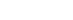# JavaScript mathematical curve – interlocking spiral

Time：2021-11-5

## Introduction

FollowEquiangular spiralThen try interlocking spiral.

## brief introductionstayArchimedes spiralThe general formula mentioned in, when C = – 2, is the interlocking spiral, also known as lituus curve. Roger Cotes described this curve in his book harmony mensurarum (1722). Maclaurin named the curve in 1722.

Formula description in polar coordinate system:Formula Description:

• a: Constant.
• θ ： Polar angle.

## draw

Draw the curve with canvas. The coordinate system of canvas is Cartesian coordinate system, and a transformation is needed.It can be seen from the above figure that taking a point has the following mathematical conversion relationship:

``````x = rcos(θ)
y = rsin(θ)
θ = arctan(y/x)``````

Combined with the polar coordinate system formula:This isExample, draw the main logic code:

``````function draw() {
let a = 100, angle = 0.1;
let x = 0, y = 0, points = [];
const acceleration = 0.1, circleNum = 20;
while (angle <= circleNum * 2 * Math.PI) {
const angleSqrt = Math.sqrt(angle);
x = (a / angleSqrt) * Math.cos(angle);
y = (a / angleSqrt) * Math.sin(angle);
points.push([x, y]);
angle = angle + acceleration;
}
//Method for drawing points into lines
line({ points: points});
}``````

## Long-form diagram of java reflection mechanism and its application scenarios

1. What is java reflection? In the object-oriented programming process of java, we usually need to know a Class class first, and thennew classname()way to get an object of this class. That is to say, we need to know which class we want to instantiate and which method to run when we write the code […]